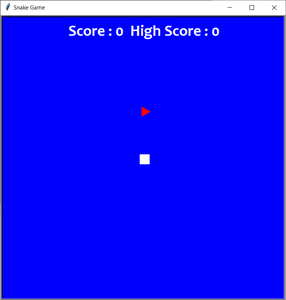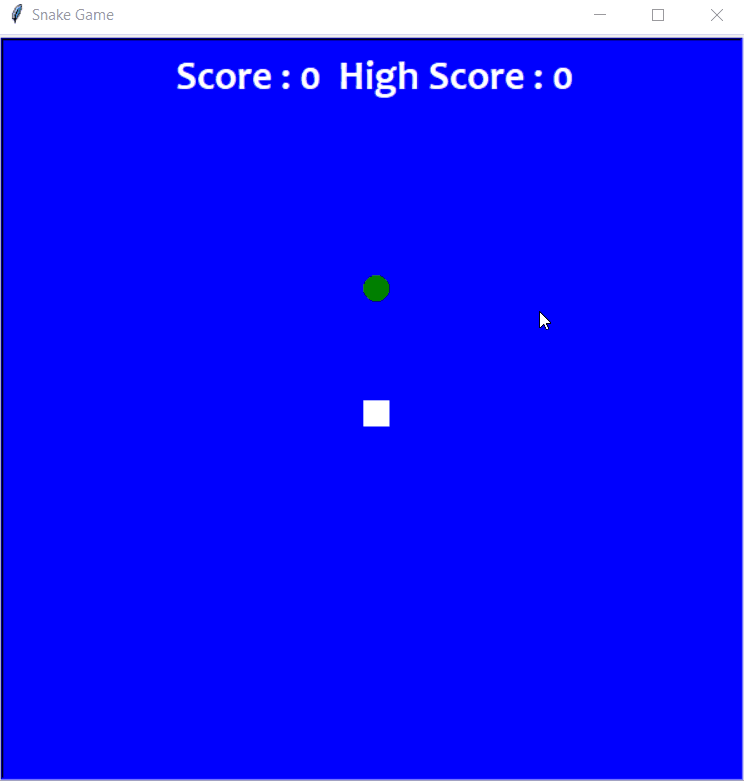# Create a Snake-Game using Turtle in Python

## Create a Snake-Game using Turtle in Python

A snake game is an arcade maze game which has been developed by Gremlin Industries and published by Sega in October 1976. It is considered to be a skillful game and has popularized among people for generations. The snake in the Snake game is controlled using the four direction buttons relative to the direction it is headed in. The player’s objective in the game is to achieve maximum points as possible by collecting food or fruits. The player loses once the snake hits the wall or hits itself.

For the python beginners, those who are interested in making something easier in your domain can definitely try this out and the module Turtle was made exactly for this purpose for the beginners to try out and can also submit as a part of the project. This program will be done in Python 3.

So, we will be creating a Python-based-game using the following modules:

• Turtle: It is a pre-installed python library that enables users to create shapes and pictures by providing them a virtual canvas.
• Time: This function is used to count the number of seconds elapsed since the epoch.
• Random: This function is used to generate random numbers in Python by using random module.

### Support

The following code can be easily done using PyCharm application which is specially made for Python programs.

Also, VSCode can be used for this program. Install Python3 from extensions of VSCode. Then, save the program in the form of your_filename.py

### Below is the step-by-step Approach to create a Snake Game using Turtle module:

Step 1: We will be importing modules into the program and giving default values for the game.

 `import` `turtle` `import` `time` `import` `random` ` ` `delay ``=` `0.1` `score ``=` `0` `high_score ``=` `0`

Step 2: Now, we will be creating the display of the game, i.e, the window screen for the game where we will create the head of the snake and food for the snake in the game and displaying the scores at the header of the game.

 `# Creating a window screen` `wn ``=` `turtle.Screen()` `wn.title(``"Snake Game"``)` `wn.bgcolor(``"blue"``)` ` ` `# the width and height can be put as user's choice` `wn.setup(width``=``600``, height``=``600``)` `wn.tracer(``0``)` ` ` ` ` `# head of the snake` `head ``=` `turtle.Turtle()` `head.shape(``"square"``)` `head.color(``"white"``)` `head.penup()` `head.goto(``0``, ``0``)` `head.direction ``=` `"Stop"` ` ` ` ` `# food in the game` `food ``=` `turtle.Turtle()` `colors ``=` `random.choice([``'red'``, ``'green'``, ``'black'``])` `shapes ``=` `random.choice([``'square'``, ``'triangle'``, ``'circle'``])` `food.speed(``0``)` `food.shape(shapes)` `food.color(colors)` `food.penup()` `food.goto(``0``, ``100``)` ` ` ` ` `pen ``=` `turtle.Turtle()` `pen.speed(``0``)` `pen.shape(``"square"``)` `pen.color(``"white"``)` `pen.penup()` `pen.hideturtle()` `pen.goto(``0``, ``250``)` `pen.write(``"Score : 0  High Score : 0"``, align``=``"center"``,` `          ``font``=``(``"candara"``, ``24``, ``"bold"``))`

Output:Step 3: Now, we will be validating the key for the snake’s movements. By clicking the keywords normally used for gaming ‘w’, ‘a’, ‘s’ and ‘d’, we can operate the snake’s movements around the screen.

 `# assigning key directions` `def` `goup():` `    ``if` `head.direction !``=` `"down"``:` `        ``head.direction ``=` `"up"` ` ` ` ` `def` `godown():` `    ``if` `head.direction !``=` `"up"``:` `        ``head.direction ``=` `"down"` ` ` ` ` `def` `goleft():` `    ``if` `head.direction !``=` `"right"``:` `        ``head.direction ``=` `"left"` ` ` ` ` `def` `goright():` `    ``if` `head.direction !``=` `"left"``:` `        ``head.direction ``=` `"right"` ` ` ` ` `def` `move():` `    ``if` `head.direction ``=``=` `"up"``:` `        ``y ``=` `head.ycor()` `        ``head.sety(y``+``20``)` `    ``if` `head.direction ``=``=` `"down"``:` `        ``y ``=` `head.ycor()` `        ``head.sety(y``-``20``)` `    ``if` `head.direction ``=``=` `"left"``:` `        ``x ``=` `head.xcor()` `        ``head.setx(x``-``20``)` `    ``if` `head.direction ``=``=` `"right"``:` `        ``x ``=` `head.xcor()` `        ``head.setx(x``+``20``)` ` ` ` ` `wn.listen()` `wn.onkeypress(goup, ``"w"``)` `wn.onkeypress(godown, ``"s"``)` `wn.onkeypress(goleft, ``"a"``)` `wn.onkeypress(goright, ``"d"``)`

Step 4: Now, lastly, we will create the gameplay where the following will be happening:

• The snake will grow its body when the snake eats the fruits.
• Giving color to the snake’s tail.
• After the fruit is eaten, the score will be counted.
• Checking for the snake’s head collisions with the body or the wall of the window screen.
• Restarting the game automatically from the start after the collision.
• The new shape and color of the fruit will be introduced every time the window is restarted.
• The score will be returned to zero and a high score will be retained until the window is not closed.
 `segments ``=` `[]` ` ` `# Main Gameplay` `while` `True``:` `    ``wn.update()` `    ``if` `head.xcor() > ``290` `or` `head.xcor() < ``-``290` `or` `head.ycor() > ``290` `or` `head.ycor() < ``-``290``:` `        ``time.sleep(``1``)` `        ``head.goto(``0``, ``0``)` `        ``head.direction ``=` `"Stop"` `        ``colors ``=` `random.choice([``'red'``, ``'blue'``, ``'green'``])` `        ``shapes ``=` `random.choice([``'square'``, ``'circle'``])` `        ``for` `segment ``in` `segments:` `            ``segment.goto(``1000``, ``1000``)` `        ``segments.clear()` `        ``score ``=` `0` `        ``delay ``=` `0.1` `        ``pen.clear()` `        ``pen.write(``"Score : {} High Score : {} "``.``format``(` `            ``score, high_score), align``=``"center"``, font``=``(``"candara"``, ``24``, ``"bold"``))` `    ``if` `head.distance(food) < ``20``:` `        ``x ``=` `random.randint(``-``270``, ``270``)` `        ``y ``=` `random.randint(``-``270``, ``270``)` `        ``food.goto(x, y)` ` ` `        ``# Adding segment` `        ``new_segment ``=` `turtle.Turtle()` `        ``new_segment.speed(``0``)` `        ``new_segment.shape(``"square"``)` `        ``new_segment.color(``"orange"``)  ``# tail colour` `        ``new_segment.penup()` `        ``segments.append(new_segment)` `        ``delay ``-``=` `0.001` `        ``score ``+``=` `10` `        ``if` `score > high_score:` `            ``high_score ``=` `score` `        ``pen.clear()` `        ``pen.write(``"Score : {} High Score : {} "``.``format``(` `            ``score, high_score), align``=``"center"``, font``=``(``"candara"``, ``24``, ``"bold"``))` `    ``# Checking for head collisions with body segments` `    ``for` `index ``in` `range``(``len``(segments)``-``1``, ``0``, ``-``1``):` `        ``x ``=` `segments[index``-``1``].xcor()` `        ``y ``=` `segments[index``-``1``].ycor()` `        ``segments[index].goto(x, y)` `    ``if` `len``(segments) > ``0``:` `        ``x ``=` `head.xcor()` `        ``y ``=` `head.ycor()` `        ``segments[``0``].goto(x, y)` `    ``move()` `    ``for` `segment ``in` `segments:` `        ``if` `segment.distance(head) < ``20``:` `            ``time.sleep(``1``)` `            ``head.goto(``0``, ``0``)` `            ``head.direction ``=` `"stop"` `            ``colors ``=` `random.choice([``'red'``, ``'blue'``, ``'green'``])` `            ``shapes ``=` `random.choice([``'square'``, ``'circle'``])` `            ``for` `segment ``in` `segments:` `                ``segment.goto(``1000``, ``1000``)` `            ``segment.clear()` ` ` `            ``score ``=` `0` `            ``delay ``=` `0.1` `            ``pen.clear()` `            ``pen.write(``"Score : {} High Score : {} "``.``format``(` `                ``score, high_score), align``=``"center"``, font``=``(``"candara"``, ``24``, ``"bold"``))` `    ``time.sleep(delay)` ` ` `wn.mainloop()`

Below the complete program based on the above approach:

 `# import required modules` `import` `turtle` `import` `time` `import` `random` ` ` `delay ``=` `0.1` `score ``=` `0` `high_score ``=` `0` ` ` ` ` ` ` `# Creating a window screen` `wn ``=` `turtle.Screen()` `wn.title(``"Snake Game"``)` `wn.bgcolor(``"blue"``)` `# the width and height can be put as user's choice` `wn.setup(width``=``600``, height``=``600``)` `wn.tracer(``0``)` ` ` `# head of the snake` `head ``=` `turtle.Turtle()` `head.shape(``"square"``)` `head.color(``"white"``)` `head.penup()` `head.goto(``0``, ``0``)` `head.direction ``=` `"Stop"` ` ` `# food in the game` `food ``=` `turtle.Turtle()` `colors ``=` `random.choice([``'red'``, ``'green'``, ``'black'``])` `shapes ``=` `random.choice([``'square'``, ``'triangle'``, ``'circle'``])` `food.speed(``0``)` `food.shape(shapes)` `food.color(colors)` `food.penup()` `food.goto(``0``, ``100``)` ` ` `pen ``=` `turtle.Turtle()` `pen.speed(``0``)` `pen.shape(``"square"``)` `pen.color(``"white"``)` `pen.penup()` `pen.hideturtle()` `pen.goto(``0``, ``250``)` `pen.write(``"Score : 0  High Score : 0"``, align``=``"center"``,` `          ``font``=``(``"candara"``, ``24``, ``"bold"``))` ` ` ` ` ` ` `# assigning key directions` `def` `goup():` `    ``if` `head.direction !``=` `"down"``:` `        ``head.direction ``=` `"up"` ` ` ` ` `def` `godown():` `    ``if` `head.direction !``=` `"up"``:` `        ``head.direction ``=` `"down"` ` ` ` ` `def` `goleft():` `    ``if` `head.direction !``=` `"right"``:` `        ``head.direction ``=` `"left"` ` ` ` ` `def` `goright():` `    ``if` `head.direction !``=` `"left"``:` `        ``head.direction ``=` `"right"` ` ` ` ` `def` `move():` `    ``if` `head.direction ``=``=` `"up"``:` `        ``y ``=` `head.ycor()` `        ``head.sety(y``+``20``)` `    ``if` `head.direction ``=``=` `"down"``:` `        ``y ``=` `head.ycor()` `        ``head.sety(y``-``20``)` `    ``if` `head.direction ``=``=` `"left"``:` `        ``x ``=` `head.xcor()` `        ``head.setx(x``-``20``)` `    ``if` `head.direction ``=``=` `"right"``:` `        ``x ``=` `head.xcor()` `        ``head.setx(x``+``20``)` ` ` ` ` `         ` `wn.listen()` `wn.onkeypress(goup, ``"w"``)` `wn.onkeypress(godown, ``"s"``)` `wn.onkeypress(goleft, ``"a"``)` `wn.onkeypress(goright, ``"d"``)` ` ` `segments ``=` `[]` ` ` ` ` ` ` `# Main Gameplay` `while` `True``:` `    ``wn.update()` `    ``if` `head.xcor() > ``290` `or` `head.xcor() < ``-``290` `or` `head.ycor() > ``290` `or` `head.ycor() < ``-``290``:` `        ``time.sleep(``1``)` `        ``head.goto(``0``, ``0``)` `        ``head.direction ``=` `"Stop"` `        ``colors ``=` `random.choice([``'red'``, ``'blue'``, ``'green'``])` `        ``shapes ``=` `random.choice([``'square'``, ``'circle'``])` `        ``for` `segment ``in` `segments:` `            ``segment.goto(``1000``, ``1000``)` `        ``segments.clear()` `        ``score ``=` `0` `        ``delay ``=` `0.1` `        ``pen.clear()` `        ``pen.write(``"Score : {} High Score : {} "``.``format``(` `            ``score, high_score), align``=``"center"``, font``=``(``"candara"``, ``24``, ``"bold"``))` `    ``if` `head.distance(food) < ``20``:` `        ``x ``=` `random.randint(``-``270``, ``270``)` `        ``y ``=` `random.randint(``-``270``, ``270``)` `        ``food.goto(x, y)` ` ` `        ``# Adding segment` `        ``new_segment ``=` `turtle.Turtle()` `        ``new_segment.speed(``0``)` `        ``new_segment.shape(``"square"``)` `        ``new_segment.color(``"orange"``)  ``# tail colour` `        ``new_segment.penup()` `        ``segments.append(new_segment)` `        ``delay ``-``=` `0.001` `        ``score ``+``=` `10` `        ``if` `score > high_score:` `            ``high_score ``=` `score` `        ``pen.clear()` `        ``pen.write(``"Score : {} High Score : {} "``.``format``(` `            ``score, high_score), align``=``"center"``, font``=``(``"candara"``, ``24``, ``"bold"``))` `    ``# Checking for head collisions with body segments` `    ``for` `index ``in` `range``(``len``(segments)``-``1``, ``0``, ``-``1``):` `        ``x ``=` `segments[index``-``1``].xcor()` `        ``y ``=` `segments[index``-``1``].ycor()` `        ``segments[index].goto(x, y)` `    ``if` `len``(segments) > ``0``:` `        ``x ``=` `head.xcor()` `        ``y ``=` `head.ycor()` `        ``segments[``0``].goto(x, y)` `    ``move()` `    ``for` `segment ``in` `segments:` `        ``if` `segment.distance(head) < ``20``:` `            ``time.sleep(``1``)` `            ``head.goto(``0``, ``0``)` `            ``head.direction ``=` `"stop"` `            ``colors ``=` `random.choice([``'red'``, ``'blue'``, ``'green'``])` `            ``shapes ``=` `random.choice([``'square'``, ``'circle'``])` `            ``for` `segment ``in` `segments:` `                ``segment.goto(``1000``, ``1000``)` `            ``segment.clear()` ` ` `            ``score ``=` `0` `            ``delay ``=` `0.1` `            ``pen.clear()` `            ``pen.write(``"Score : {} High Score : {} "``.``format``(` `                ``score, high_score), align``=``"center"``, font``=``(``"candara"``, ``24``, ``"bold"``))` `    ``time.sleep(delay)` ` ` `wn.mainloop()`Demonstrating the final game displaying everything written in code

Last Updated on March 17, 2022 by admin

## Instance method in PythonInstance method in Python

Instance method in Python   A class is a user-defined blueprint or prototype from which

## Python statistics variance()Python statistics variance()

Python statistics | variance() Statistics module provides very powerful tools, which can be used to compute

## Simple registration form using Python TkinterSimple registration form using Python Tkinter

Simple registration form using Python Tkinter Python provides the Tkinter toolkit to develop GUI applications.

## Python | Generate random numbers within a given range and store in a listPython | Generate random numbers within a given range and store in a list

Python | Generate random numbers within a given range and store in a list Given

## Normal Equation in Linear RegressionNormal Equation in Linear Regression

ML | Normal Equation in Linear Regression Normal Equation is an analytical approach to Linear Regression

## Interquartile Range and Quartile Deviation using NumPy and SciPyInterquartile Range and Quartile Deviation using NumPy and SciPy

Interquartile Range and Quartile Deviation using NumPy and SciPy Quartiles : A quartile is a

## sys.stdout.write in Pythonsys.stdout.write in Python

sys.stdout.write in Python This is a built-in Python module that contains parameters specific to the

## Play sound in PythonPlay sound in Python

Play sound in Python In this article, we will see how to play sound in